# Modified PSFEx Profile and the Outer Wings of Stars

Page author and contact: Rongpu Zhou

Starting with DR9, we use an extended PSF model to subtract the extended wings of bright stars from DECam images. The model is symmetric (which is a good approximation for DECam images), and is a linear combination of two components:

1. A flexible inner PSF, which is a Moffat profile fit to the PSFEx image.

2. A fixed outer PSF, which is either a power law (for $g\hbox{-}$ and $r\hbox{-}\mathrm{bands}$) or a Moffat profile (for $z\hbox{-}\mathrm{band}$).

## The Inner PSF Profile

The inner PSF component is a Moffat profile:

• $f_{\mathrm{Moffat}} = \frac{\beta-1}{\pi \alpha^2} \left[1 + \frac{r}{\alpha^2}\right]^{-\beta}$

This formula gives a total flux of unity by definition. Its two free parameters, $\alpha$ and $\beta$, are obtained by fitting to the PSFEx image of each CCD. Only PSFEx pixels between 1.8 and 5 arcsec in radius are used in the fit.

The size of the DECam PSFEx image is only ~8.1 arcsec in radius, too small for implementing the extended PSF model. Nevertheless, we modify the the PSFEx image for the following reasons:

1. It removes discontinuities when we subtract the outer wings of bright stars from the images

2. The noisy parts of the PSFEx images are replaced by a noiseless model PSF. This is more of a cosmetic improvement and it should not affect the Tractor fitting of unsaturated stars or faint galaxies.

Here are illustrations of the weights for patching the PSFEx image and the weights of the stellar halo subtraction: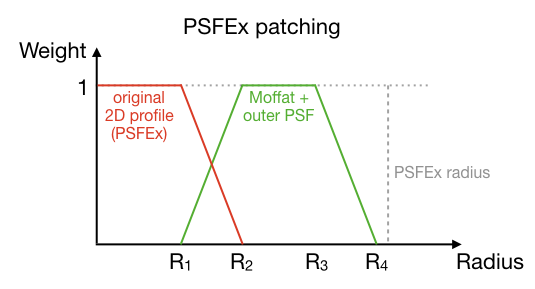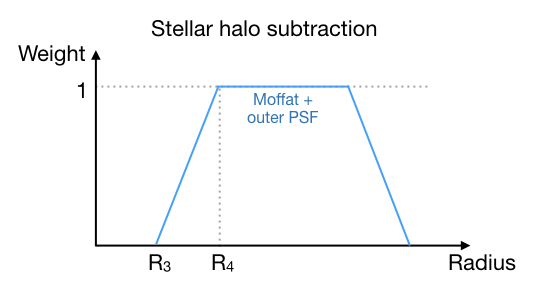The (tentative) $R$ values are $R_1, R_2, R_3, R_4 = 5.0, 6.0, 7.0, 8.0\,(\mathrm{arcsec}$). The transition radii $R_1$ and $R_2$ are chosen so that beyond radius $R_1$ there is little asymmetry in the PSFEx model (i.e. the symmetric Moffat profile is a good approximation). For reference, the size of the PSFEx images is ~8.1 arcsec in radius ($63 \times 63$ with pixel size $0.262\,\mathrm{arcsec}$) for DECam and Mosaic, and ~6.8 arcsec ($31 \times 31$ with pixel size $0.454\,\mathrm{arcsec}$) for 90Prime.

In the patched PSFEx image (the first eigen-image), the crossover between $R_1$ and $R_2$ marks the transition between the PSFEx image and the model PSF. The flux gradually approaches zero between $R_3$ and $R_4$, and is strictly zero beyond $R_4$. For the other eigenimages, the values for each pixel gradually approach zero between $R_1$ and $R_2$ (i.e. the red curve in the above plot), and they are strictly zero beyond $R_2$. To preserve continuity, the outer PSF component is included for PSFEx patching, even though it's not used in the fitting; it contributes a negligible fraction of the flux in the PSFEx image.

Here is an example of the Moffat profile fit to the PSFEx image, and the corresponding original and patched PSFEx image: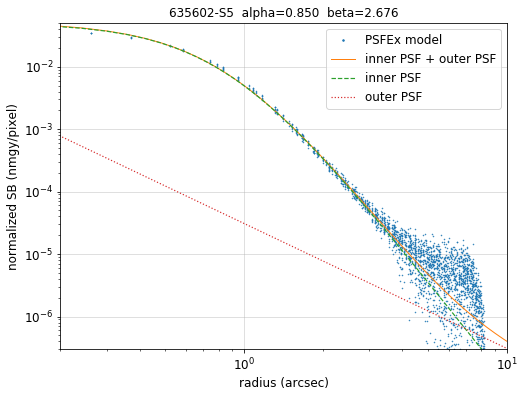## The Outer PSF Profile

For $g\hbox{-}$, $r\hbox{-}$ and $i\hbox{-}\mathrm{bands}$, the outer PSF profile is a fixed power law (normalized to a 22.5 magnitude star, in units of $\mathrm{nanomaggies}/\mathrm{arcsec}^2$):

• $f_g = 0.00045 r^{-2}$

• $f_r = 0.00033 r^{-2}$

• $f_i = 0.00033 r^{-2}$

For $z\hbox{-}\mathrm{band}$, the outer PSF is a weighted Moffat profile. For most CCDs, the Moffat parameters (with radius in arcsec and surface brightness in $\mathrm{nanomaggies}/\mathrm{arcsec}^2$) and the weights are (for a 22.5 magnitude star):

• $[\alpha, \beta, weight] = [17.650, 1.7, 0.0145]$

However, a small subset of DECam CCDs (N20, S8, S10, S18, S21 and S27) have a more compact outer PSF in the $z\hbox{-}\mathrm{band}$, which is characterized using:

• $[\alpha, \beta, weight] = [16, 2.3, 0.0095]$

## The Combined PSF model

Below is an example of the model PSF profile in $g\hbox{-}\mathrm{band}$, with the pixel fluxes around a bright star overplotted. The inner PSF's are obtained by fitting the PSFEx image, and the outer PSF profile is fixed and normalized to a DECam magnitude predicted from Gaia magnitudes and colors. Also included are the corresponding original and halo-subtracted images.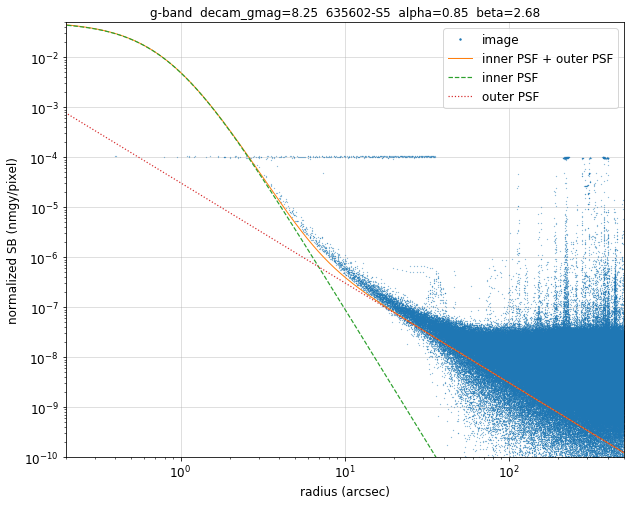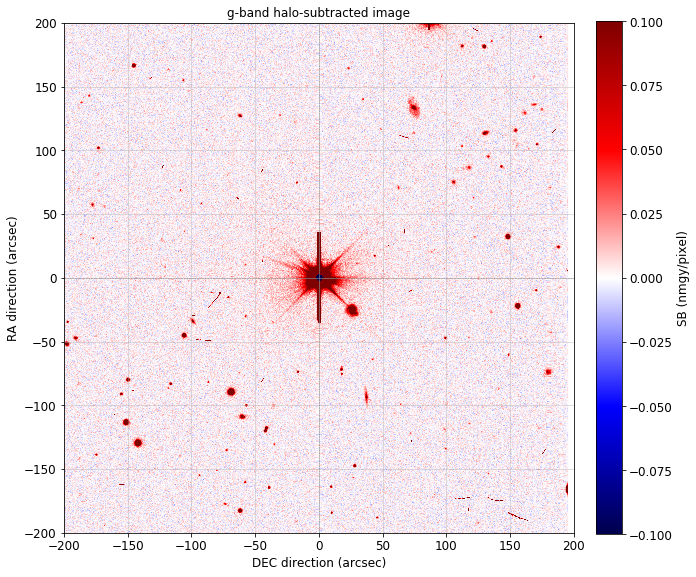## CCD-to-CCD variations in $z\hbox{-}\mathrm{band}$

A surprising finding from this work is that a small subset of DECam CCDs have different PSFs compared to the other CCDs in the $z\hbox{-}\mathrm{band}$. These outlier CCDs have consistently more compact PSFs at the $r>\sim20\,\mathrm{arcsec}$ scale, and they can be approximated by a different Moffat profile. In DECaLS, this difference only shows up in the $z\hbox{-}\mathrm{band}$ images (although it's possible that such CCD-to-CCD variations might also appear in, e.g., $i\hbox{-}\mathrm{band}$ or $Y\hbox{-}\mathrm{band}$ images from DECam). The cause of these variations is unclear.

## File locations

The PSFEx files used for the above analysis are available in the following directories at NERSC:

• Original PSF: /global/cfs/cdirs/cosmo/work/legacysurvey/dr9-archive/unpatched-psfex

• Patched PSF: /global/cfs/cdirs/cosmo/data/legacysurvey/dr9/calib/psfex

The equivalent files used to modify the PSF for DR10 are available at:

• Original PSF: /global/cfs/cdirs/cosmo/work/legacysurvey/dr10/attic/TO-ARCHIVE/calib/unpatched-psfex.tgz (in a gzipped tar archive)

• Patched PSF: /global/cfs/cdirs/cosmo/data/legacysurvey/dr10/calib/psfex

## Code

The code used to analyze the DECam PSF for the Legacy Surveys is: